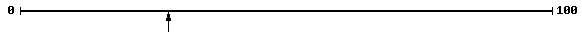• ## Accessibility

Open the accessibility toolbar to change fonts and contrast, access a dictionary, use a ruler and more

In this worksheet estimate the number the arrow points to on a number line to 100.

Example

Estimate the number the arrow is pointing to.Divide the line up into halves, quarters, etc and then take a guess at where you think the arrow is.

The half way point would be 50.  The quarters would be at 25, 50, 75.

You could try tenths as well.

Here the answer is 28, but answers of  26, 27, 28, 29, 30 would be accepted.

10 questions